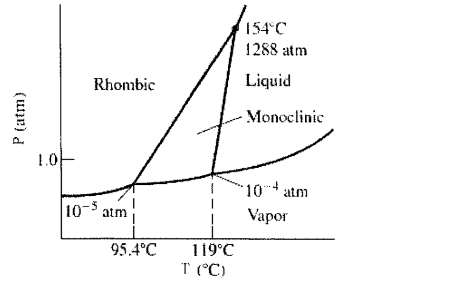# Problem: Sulfur can exist as a gas, a liquid, or as one of two solid states: rhombic and monoclinic. A phase diagram for sulfur is shown below: a. What is (are) the thermodynamically-stable phase(s) of sulfur at room temperature and pressure (i.e. 1.0 atm at 25°C)?     b. Which state of sulfur is more dense: rhombic, monoclinic, or liquid?

🤓 Based on our data, we think this question is relevant for Professor TBA's class at NYU.

###### Problem Details

Sulfur can exist as a gas, a liquid, or as one of two solid states: rhombic and monoclinic. A phase diagram for sulfur is shown below:

a. What is (are) the thermodynamically-stable phase(s) of sulfur at room temperature and pressure (i.e. 1.0 atm at 25°C)?

b. Which state of sulfur is more dense: rhombic, monoclinic, or liquid?What scientific concept do you need to know in order to solve this problem?

Our tutors have indicated that to solve this problem you will need to apply the Phase Diagram concept. You can view video lessons to learn Phase Diagram. Or if you need more Phase Diagram practice, you can also practice Phase Diagram practice problems.

What is the difficulty of this problem?

Our tutors rated the difficulty ofSulfur can exist as a gas, a liquid, or as one of two solid ...as medium difficulty.

How long does this problem take to solve?

Our expert Chemistry tutor, Sabrina took 1 minute and 11 seconds to solve this problem. You can follow their steps in the video explanation above.

What professor is this problem relevant for?

Based on our data, we think this problem is relevant for Professor TBA's class at NYU.